# NCERT Solutions for Class 8 Maths Chapter 6 Square and Square Roots Ex 6.4

These NCERT Solutions for Class 8 Maths Chapter 6 Square and Square Roots Ex 6.4 Questions and Answers are prepared by our highly skilled subject experts.

## NCERT Solutions for Class 8 Maths Chapter 6 Square and Square Roots Exercise 6.4

Question 1.
Find the square root of each of the following numbers by division method.
(i) 2304
(ii) 4489
(iii) 3481
(iv) 529
(v) 3249
(vi) 1369
(vii) 5776
(viii) 7921
(ix) 576
(x) 1024
(xi) 3136
(xii) 900
Solution:
(i) 2304
∴ √2304 = 48(ii) 4489
∴ √4489 = 67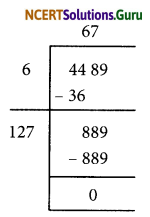(iii) 3481
∴ √3481 = 59(iv) 529
∴ √529 = 23(v) 3249
∴ √3249 = 57(vi) 1369
∴ √1369 = 37(vii) 5776
∴ √5776 = 76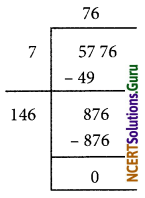(viii) 7921
∴ √7921 = 89(ix) 576
∴ √576 = 24(x) 1024
∴ √1024 = 32(xi) 3136
∴ √3136 = 56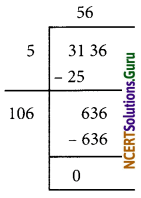(xii) 900
∴ √900 = 30Question 2.
Find the number of digits in the square root of each of the following numbers. (without any calculation).
(i) 64
(ii) 144
(iii) 4489
(iv) 27225
(v) 390625
Solution:
If n stands fnr nnmher of dibits in the given number then
(i) For 64
Here, n = 2 (even number)
Number of digits in the square root = $$\frac{n}{2}=\frac{2}{2}=1$$

(ii) For 144
Here, n = 3 (odd number)
Number of digits in the, square root = $$\frac{n+1}{2}=\frac{3+4}{2}=\frac{4}{2}=2$$

(iii) For 4489
Here, n = 4 (even number)
Number of digits in the square root = $$\frac{\mathrm{n}}{2}=\frac{4}{2}=2$$(iv) For 27225
Here, n = 5 (odd number)
Number of digits in the square root = $$\frac{\mathrm{n}+1}{2}=\frac{5+1}{2}=\frac{6}{2}=3$$

(v) For 390625
Here, n = 6 (even number)
Number of digits in the square root = $$\frac{n}{2}=\frac{6}{2}=3$$

Question 3.
Find the square root of the following decimal numbers.
(i) 2.56
(ii) 7.29
(iii) 51.84
(iv) 42.25
(v) 31.36
Solution:
(i) 2.56
∴ √2.56 = 1.6(ii) 7.29
∴ √7.29 = 2.7(iii) 51.84
∴ √51.84 = 7.2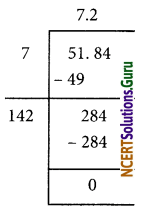(iv) 42.25
∴ √42.25 = 6.5(v) 31.36
∴ √31.36 = 5.6Question 4.
Find the least number which must be subtracted from each of the following numbers so as to get a perfect square. Also, find the square root of the perfect square so obtained.
(i) 402
(ii) 1989
(iii) 3250
(iv) 825
(v) 4000
Solution:
(i) 402
The required least number to be subtracted from 402 is 2.
∴ 402 – 2 = 400
∴ √400 = 20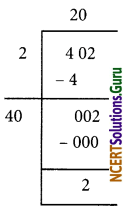(ii) 1989
The remainder is 53
∴ The required least numbers to be subtracted from the given number is 53.
1989 – 53 = 1936
∴ √1936 = 44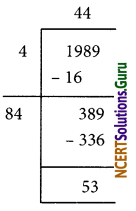(iii) 3250
The remainder is 1
∴ The required least number to be subtracted from the given number is 1.
3250 – 1 = 3249
∴ √3249 = 57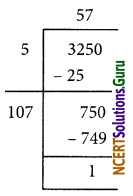(iv) 825
The remainder is 41
The required least number to be subtracted from the given number is 41.
825 – 41 = 784
∴ √784 = 28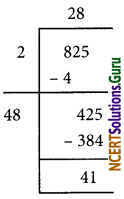(v) 4000
The remainder is 31
The required least number to be subtracted from the given number is 31.
4000 – 31 = 3969
∴ √3969 = 63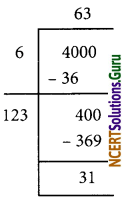Question 5.
Find the least number which must be added to each of the following numbers so as to get a perfect square. Also, find the square root of the perfect square, so obtained.
(i) 525
(ii) 1750
(iii) 252
(iv) 1825
(v) 6412
Solution:
(i) 525
The remainder is 41.
i.e. 525 > 222 and the next perfect square number is 232 = 529
The required number to be added = 232 – 525
= 529 – 525
= 4
Hence, 4 should be added to 525 to get a perfect square
number.
525 + 4 = 529
∴ √529 = 23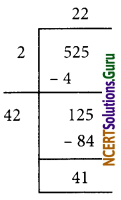(ii) 1750
The remainder is 69.
1750 > 412 and the next perfect square number is 422 = 1764
The required number to be added = 422 – 1750
= 1764 – 1750
= 14
The next perfect square number = 1750 + 14 = 1764
∴ √1764 = 42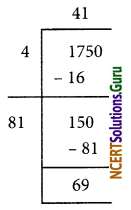(iii) 252
The remainder is 27.
This shows that 152 < 252
The next perfect square number is 162 = 256.
The required number to be added = 162 – 252
= 256 – 252
= 4
The perfect square number = 252 + 4 = 256
∴ √256 = 16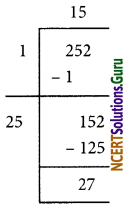(iv) 1825
The remainder is 61.
This shows that 422 < 1825
The next perfect square is 432 = 1849
Hence, the required number to be added = 432 – 1825
= 1849 – 1825
= 24
The perfect square number so obtained 1825 + 24 = 1849
Hence, √1849 = 43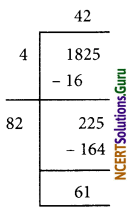(v) 6412
The remainder is 12.
This shows that 802 < 6412
The next perfect square number is 812 = 6561
The required number to be added = 6561 – 6412 = 149
The perfect square number = 6412 + 149 = 6561
Hence, √6561 = 81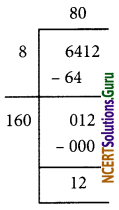Question 6.
Find the length of the side of a square where area is 441m2.
Solution:
Let x be the side of a square.
∴ Area of square = x2
Given, Area of square = 441
x2 = 441
⇒ x = √441 = 21
So, length of the side of the square = 21 m.Question 7.
In a right triangle ABC, ∠B = 90°.
(a) If AB = 6 cm, BC = 8 cm, find AC.
(b) If AC = 13 cm, BC = 5 cm, find AB.
Solution:
(a) In the right triangle ABC, ∠B = 90°By Pythagoras Theorem,
AC2 = AB2 + BC2
⇒ AC2 = 62 + 82
⇒ AC2 = 36 + 64
⇒ AC2 = 100
⇒ AC = √100 = 10
∴ Length of AC = 10 cm(b) In the right triangle ABC, ∠B = 90°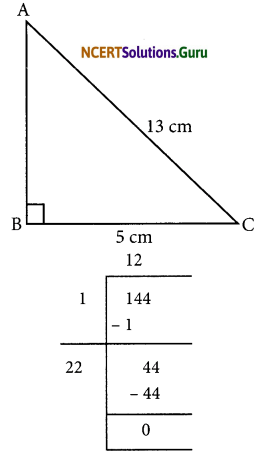By Pythagoras theorem,
AC2 = AB2 + BC2
⇒ AB2 = AC2 – BC2
⇒ AB2 = 132 – 52
⇒ AB2 = 169 – 25
⇒ AB2 = 144
⇒ AB = √144 = 12
∴ Length of AB = 12 cm

Question 8.
A gardener has 1000 plants. He wants to plant these in such a way that the number of rows and the number of columns remain same. Find the minimum number of plants he needs more for this.
Solution:
Let the number of rows be ‘x’
The number of columns is x
The number of plants = x × x = x2
which is a perfect square
Let us find out the square root of 1000 by division method
The remainder is 39
This shows that 312 < 1000
The next perfect square number is 322 = 1024
Minimum number of plants he needs besides 100 plants = 322 – 1000
= 1024 – 1000
= 24Question 9.
There are 500 children in a school. For a P.T. drill, they have to stand in such a manner that the number of rows is equal to number of columns. How many children would be left out in this arrangement.
Solution:
Let the number of rows be x and the number of columns is x
∴ Number of children = x × x = x2
which is a perfect square
∴ 500 = x2
The remainder is 16.
Number of children left out in this arrangement = 16.error: Content is protected !!